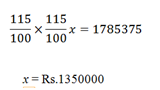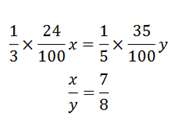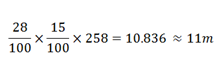# RRB Practice Questions | Mathematics – Day-07

Dear Aspirants,

Mathematics is a complex web. So only most of the applicant’s thought is RRB Mathematics questions are tough to work out. It’s really not, to score the maximum mark in this section you need of regular practice on RRB Maths every day.

Start to practice Quiz for RRB Mathematics section and reveal the answers with detailed explanation. We have taken much care on the explanation part that to understand the RRB Mathematics questions easily. Take RRB Mathematics quiz regularly to perform better on  RRB Maths section without any hurdle.

[WpProQuiz 5626]

1) Sanjay earns an annual income Rs.1785375 at present. His company increases his income at 15% per annum every year. What was Sanjay’s annual income 2 years before?

a) Rs.1350000

b) Rs.1355000

c) Rs.1450000

d) Rs.1550000

2) The growth of a production of company A between 2000 and 2001 was 20% and that of between 2001 and 2002 was 80%. What was the percentage growth between 2000 and 2002?

a) 120%

b) 116%

c) 100%

d) 124%

3) A shopkeeper employed a man for monthly salary of Rs.1200. In addition to it, he agreed to pay him a commission of 12% on the monthly sale. How much sale in rupees should the man do if he wants his monthly salary as Rs.7200?

a) Rs.33000

b) Rs.45000

c) Rs.60000

d) Rs.50000

4) Ajay has an average of 54% on his first 6 exams. How much should he take on his seventh exam to obtain an average of 60% on the seventh exam?

a) 96%

b) 80%

c) 52%

d) Cannot be determined

5) Rohan invests 12% of his monthly salary i.e., Rs.6024 in mutual funds. Later he invests 15% of his monthly salary on pension schemes also he invests 9% of his salary in Insurance Policies. What is the total amount invested by Rohan?

a) Rs.18072

b) Rs.15088

c) Rs.18100.45

d) Rs.16542

6) Mr. Sharma’s salary was increased by 40% in a month and subsequently decreased by 50%. How much percent did he lose/gain?

a) Gain 40%

b) Loss 30%

c) Gain 25%

d) Loss 32%

7) In a regional election between three candidates, A got 23% of the total valid votes and C got 54% of the total valid votes. 20% of the total votes were invalid. If the total votes are 16000. What is the number of valid votes the remaining person got?

a) 3680

b) 3200

c) 2944

d) 736

8) One – third of 24% of a number is equal to one-fifth of 35% of another number. What is the ratio of the two numbers?

a) 8:7

b) 8:9

c) 7:9

d) None of these

9) A man climbed 72 % of the height of a pole in one hour and in the next hour he covered 15% of the remaining height. If the height of the pole was 258m, then what was the distance covered by him in the second hour?

a) 9 m

b) 11 m

c) 12 m

d) 14 m

10) Anand spends 12% of his monthly income on house rent, 22% on food. Of the remaining salary he spends 18% on entertainment and 22% for travelling expense. He is now left with Rs.10920. What is his monthly salary?

a) Rs.38000

b) Rs.42000

c) Rs.48000

d) Rs.52000Total growth percentage = 20 + 60 + (20 × 60)/100 = 116%

7200 – 1200 = 6000

12% = 6000

100% =6000 × 100 / 12 = Rs.50000

Ajay gets 60% of marks out of the total marks in 7 subjects. We could find the percentage with using total marks of the exam. Here there is no data about the total marks. So we cannot conclude.

12% → 6024

12+15+9 = 36%

36% = 6024 × 36 / 12 = Rs.18072

Let the salary be Rs.100

New salary = 100 × (140/100) × (50/100) = Rs.70

Percentage of loss = 30%

100 % → 16000

80% → 12800

Votes of A and C got 23% + 54% = 77%

100 % → 12800

23% → 2944 valid votes got by the remaining personLet total salary = 100%

House rent = 12%

Food = 22%

Remaining = 66%

Then he spends on Entertainment – 18%

Travelling expenses – 22%

Rest = 66% – 40% = 26%

26% →10920

100% → 42000